Test: Z-Transform

# Test: Z-Transform

Test Description

## 10 Questions MCQ Test Topicwise Question Bank for Electrical Engineering | Test: Z-Transform

Test: Z-Transform for Electrical Engineering (EE) 2023 is part of Topicwise Question Bank for Electrical Engineering preparation. The Test: Z-Transform questions and answers have been prepared according to the Electrical Engineering (EE) exam syllabus.The Test: Z-Transform MCQs are made for Electrical Engineering (EE) 2023 Exam. Find important definitions, questions, notes, meanings, examples, exercises, MCQs and online tests for Test: Z-Transform below.
Solutions of Test: Z-Transform questions in English are available as part of our Topicwise Question Bank for Electrical Engineering for Electrical Engineering (EE) & Test: Z-Transform solutions in Hindi for Topicwise Question Bank for Electrical Engineering course. Download more important topics, notes, lectures and mock test series for Electrical Engineering (EE) Exam by signing up for free. Attempt Test: Z-Transform | 10 questions in 30 minutes | Mock test for Electrical Engineering (EE) preparation | Free important questions MCQ to study Topicwise Question Bank for Electrical Engineering for Electrical Engineering (EE) Exam | Download free PDF with solutions
 1 Crore+ students have signed up on EduRev. Have you?
Test: Z-Transform - Question 1

### if, x(z) iswith  |z| >1, then what is the corresponding x(n)?

Test: Z-Transform - Question 2

### For which of the following x[n] cannot find z-transform x(z).

Test: Z-Transform - Question 3

### The z-transform of a signal is given by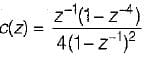Its final valve is

Test: Z-Transform - Question 4

Which one of the following represents the impulse response of a system defined by H(z) = z-m

Test: Z-Transform - Question 5

The z-transform of the time function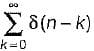is

Test: Z-Transform - Question 6

The z-transform of the system is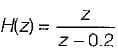if the ROC is |z| < 0.2, then the impulse response of the system is

Test: Z-Transform - Question 7

If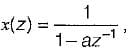the two discrete time signal

Test: Z-Transform - Question 8

lf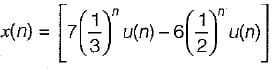The ROC for the above discrete time signal is

Test: Z-Transform - Question 9

A stable and causal system is described by the difference equation,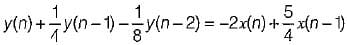The impulses response of the system is

Test: Z-Transform - Question 10

A linear discrete time system has the characteristics equation, z3 - 0.81 z = 0, the system is

## Topicwise Question Bank for Electrical Engineering

207 tests
 Use Code STAYHOME200 and get INR 200 additional OFF Use Coupon Code